##### The Japanese Bonsai specialist
Direct order Contact Help / Services Newsletter# Pinus thunbergii 'kotobuki'

Kuro matsu kotobuki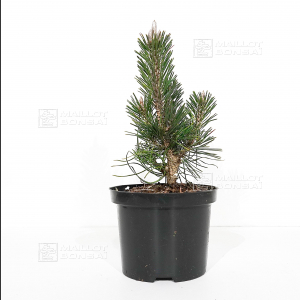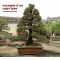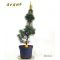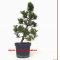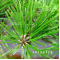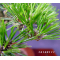ref. : 10324,30

This item is temporarily unavailable

###### Description

Tree produced by grafting.

Delivered in a plastic pot: Ø 16 * 12cm.

Height of the tree only: 20-30 cm.

Trunk Ø 1 cm.

A variety of rare black pine, pinus thunbergii 'Kotobuki' is an ideal species to turn into a bonsai thanks to its thin needles. The photos show the kind of fine tree it can grow into after many years. It can be repotted into a non-enamelled pot once it's had time to develop. Potted in 09-2021 don't touch root ball before 09-2022.

#thunbergii 4 #kotobuki 3.8 #pinus 3.5 #into 3.4 #tree 3.4 #trees 2.5 #young 2.5 #enamelled 1.9 #delivered 1.9 #repotted 1.8

Formule
(( ROUND((CHAR_LENGTH(b.article_nom)-CHAR_LENGTH(REPLACE(b.article_nom, 'thunbergii', '')))/LENGTH('thunbergii')) + ROUND((CHAR_LENGTH(b.article_description)-CHAR_LENGTH(REPLACE(b.article_description, 'thunbergii', '')))/LENGTH('thunbergii')) ) * 4) + (( ROUND((CHAR_LENGTH(b.article_nom)-CHAR_LENGTH(REPLACE(b.article_nom, 'kotobuki', '')))/LENGTH('kotobuki')) + ROUND((CHAR_LENGTH(b.article_description)-CHAR_LENGTH(REPLACE(b.article_description, 'kotobuki', '')))/LENGTH('kotobuki')) ) * 3.8) + (( ROUND((CHAR_LENGTH(b.article_nom)-CHAR_LENGTH(REPLACE(b.article_nom, 'pinus', '')))/LENGTH('pinus')) + ROUND((CHAR_LENGTH(b.article_description)-CHAR_LENGTH(REPLACE(b.article_description, 'pinus', '')))/LENGTH('pinus')) ) * 3.5) + (( ROUND((CHAR_LENGTH(b.article_nom)-CHAR_LENGTH(REPLACE(b.article_nom, 'tree', '')))/LENGTH('tree')) + ROUND((CHAR_LENGTH(b.article_description)-CHAR_LENGTH(REPLACE(b.article_description, 'tree', '')))/LENGTH('tree')) ) * 3.4) + (( ROUND((CHAR_LENGTH(b.article_nom)-CHAR_LENGTH(REPLACE(b.article_nom, 'into', '')))/LENGTH('into')) + ROUND((CHAR_LENGTH(b.article_description)-CHAR_LENGTH(REPLACE(b.article_description, 'into', '')))/LENGTH('into')) ) * 3.4) + (( ROUND((CHAR_LENGTH(b.article_nom)-CHAR_LENGTH(REPLACE(b.article_nom, 'delivered', '')))/LENGTH('delivered')) + ROUND((CHAR_LENGTH(b.article_description)-CHAR_LENGTH(REPLACE(b.article_description, 'delivered', '')))/LENGTH('delivered')) ) * 1.9) + (( ROUND((CHAR_LENGTH(b.article_nom)-CHAR_LENGTH(REPLACE(b.article_nom, 'enamelled', '')))/LENGTH('enamelled')) + ROUND((CHAR_LENGTH(b.article_description)-CHAR_LENGTH(REPLACE(b.article_description, 'enamelled', '')))/LENGTH('enamelled')) ) * 1.9) + (( ROUND((CHAR_LENGTH(b.article_nom)-CHAR_LENGTH(REPLACE(b.article_nom, 'produced', '')))/LENGTH('produced')) + ROUND((CHAR_LENGTH(b.article_description)-CHAR_LENGTH(REPLACE(b.article_description, 'produced', '')))/LENGTH('produced')) ) * 1.8) + (( ROUND((CHAR_LENGTH(b.article_nom)-CHAR_LENGTH(REPLACE(b.article_nom, 'repotted', '')))/LENGTH('repotted')) + ROUND((CHAR_LENGTH(b.article_description)-CHAR_LENGTH(REPLACE(b.article_description, 'repotted', '')))/LENGTH('repotted')) ) * 1.8) + (( ROUND((CHAR_LENGTH(b.article_nom)-CHAR_LENGTH(REPLACE(b.article_nom, 'grafting', '')))/LENGTH('grafting')) + ROUND((CHAR_LENGTH(b.article_description)-CHAR_LENGTH(REPLACE(b.article_description, 'grafting', '')))/LENGTH('grafting')) ) * 1.8)

## Secure payment## Delivery

Our logistic partners :04 74 55 23 48
Pépinière MAILLOT-BONSAÏ
Le Bois Frazy
01990 RELEVANT - FRANCE
on appointment